## How to vlookup to return multiple values in one cell in Excel?

Normally, in Excel, when you use the VLOOKUP function, if there are multiple values to match the criteria, you just can get the first one. But, sometimes, you want to return all the corresponding values which meet the criteria into one cell as following screenshot shown, how could you solve it?

Vlookup to return multiple values into one cell with TEXTJOIN function (Excel 2019 and Office 365)

Vlookup to return multiple values into one cell with User Defined Function

Vlookup to return multiple values into one cell with a useful feature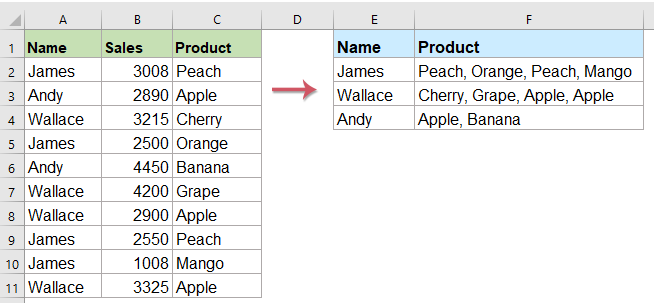#### Vlookup to return multiple values into one cell with TEXTJOIN function (Excel 2019 and Office 365)

If you have the higher version of the Excel such as Excel 2019 and Office 365, there is a new function - TEXTJOIN, with this powerful function, you can quickly vlookup and return all matching values into one cell.

###### Vlookup to return all matching values into one cell

Please apply the below formula into a blank cell where you want to put the result, then press Ctrl + Shift + Enter keys together to get the first result, and then drag the fill handle down to the cell you want to use this formula, and you will get all corresponding values as below screenshot shown:

=TEXTJOIN(",",TRUE,IF(\$A\$2:\$A\$11=E2,\$C\$2:\$C\$11,""))Note: In the above formula, A2:A11 is the lookup range contains the lookup data, E2 is the lookup value, C2:C11 is the data range that you want to return the matching values from, "," is the separator to separate the multiple records.

###### Vlookup to return all matching values without duplicates into one cell

If you want to return all matching values based on the lookup data without duplicates, the below formula may help you.

Please copy and paste the following formula into a blank cell, then press Ctrl + Shift + Enter keys together to get the first result, and then copy this formula to fill other cells, and you will get all corresponding values without the dulpicate ones as below screenshot shown:

=TEXTJOIN(",", TRUE, IF(IFERROR(MATCH(\$C\$2:\$C\$11, IF(E2=\$A\$2:\$A\$11, \$C\$2:\$C\$11, ""), 0),"")=MATCH(ROW(\$C\$2:\$C\$11), ROW(\$C\$2:\$C\$11)), \$C\$2:\$C\$11, ""))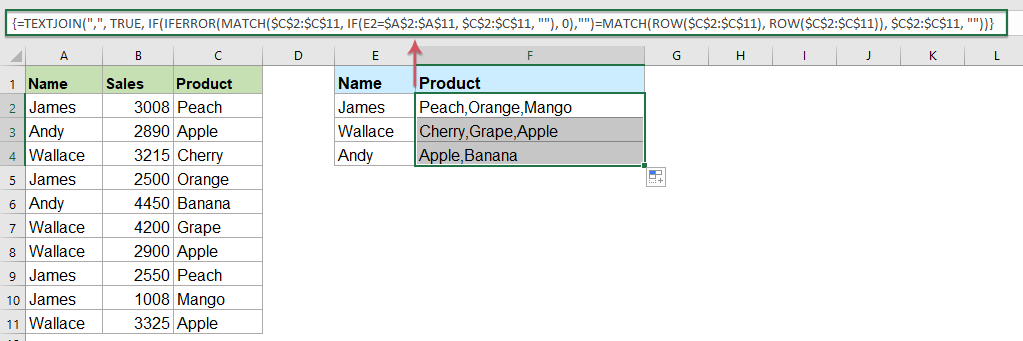Note: In the above formula, A2:A11 is the lookup range contains the lookup data, E2 is the lookup value, C2:C11 is the data range that you want to return the matching values from, "," is the separator to separate the multiple records.

#### Vlookup to return multiple values into one cell with User Defined Function

The above TEXTJOIN function is only available for Excel 2019 and Office 365, if you have other lower Excel versions, you should use some codes for finishing this task.

###### Vlookup to return all matching values into one cell

1. Hold down the ALT + F11 keys, and it opens the Microsoft Visual Basic for Applications window.

2. Click Insert > Module, and paste the following code in the Module Window.

VBA code: Vlookup to return multiple values into one cell

``````Function ConcatenateIf(CriteriaRange As Range, Condition As Variant, ConcatenateRange As Range, Optional Separator As String = ",") As Variant
'Updateby Extendoffice
Dim xResult As String
On Error Resume Next
If CriteriaRange.Count <> ConcatenateRange.Count Then
ConcatenateIf = CVErr(xlErrRef)
Exit Function
End If
For i = 1 To CriteriaRange.Count
If CriteriaRange.Cells(i).Value = Condition Then
xResult = xResult & Separator & ConcatenateRange.Cells(i).Value
End If
Next i
If xResult <> "" Then
xResult = VBA.Mid(xResult, VBA.Len(Separator) + 1)
End If
ConcatenateIf = xResult
Exit Function
End Function
``````

3. Then save and close this code, go back to the worksheet, and enter this formula: =CONCATENATEIF(\$A\$2:\$A\$11, E2, \$C\$2:\$C\$11, ", ") into a specific blank cell where you want to place the result, then drag the fill handle down to get all the corresponding values in one cell that you want, see screenshot: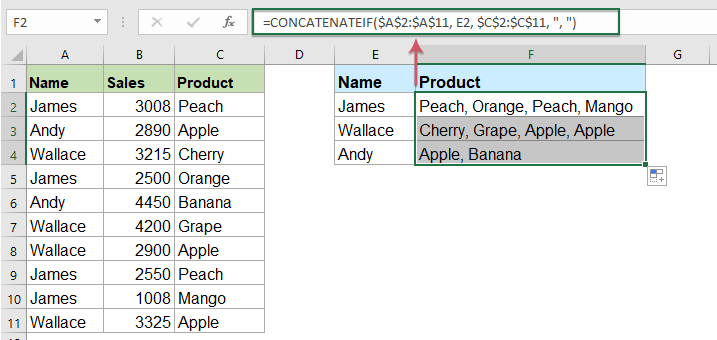Note: In the above formula, A2:A11 is the lookup range contains the lookup data, E2 is the lookup value, C2:C11 is the data range that you want to return the matching values from, "," is the separator to separate the multiple records.

###### Vlookup to return all matching values without duplicates into one cell

To ignore the duplicates in the returned matching values, please do with the below code.

1. Hold down the Alt + F11 keys to open the Microsoft Visual Basic for Applications window.

2. Click Insert > Module, and paste the following code in the Module Window.

VBA code: Vlookup and return multiple unique matched values into one cell

``````Function MultipleLookupNoRept(Lookupvalue As String, LookupRange As Range, ColumnNumber As Integer)
'Updateby Extendoffice
Dim xDic As New Dictionary
Dim xRows As Long
Dim xStr As String
Dim i As Long
On Error Resume Next
xRows = LookupRange.Rows.Count
For i = 1 To xRows
If LookupRange.Columns(1).Cells(i).Value = Lookupvalue Then
End If
Next
xStr = ""
MultipleLookupNoRept = xStr
If xDic.Count > 0 Then
For i = 0 To xDic.Count - 1
xStr = xStr & xDic.Keys(i) & ","
Next
MultipleLookupNoRept = Left(xStr, Len(xStr) - 1)
End If
End Function
``````

3. After inserting the code, then click Tools > References in the opened Microsoft Visual Basic for Applications window, and then, in the popped out References – VBAProject dialog box, check Microsoft Scripting Runtime option in the Available References list box, see screenshots: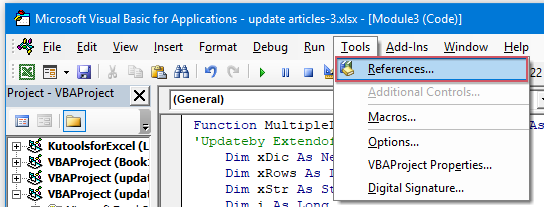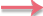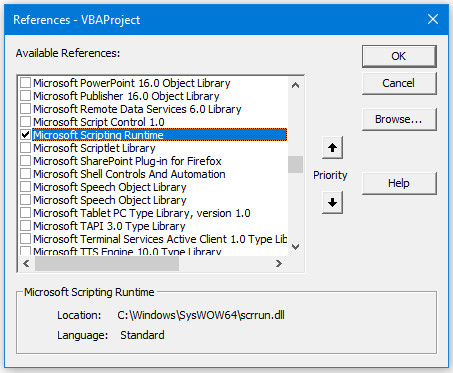4. Then click OK to close the dialog box, save and close the code window, return to the worksheet, and enter this formula: =MultipleLookupNoRept(E2,\$A\$2:\$C\$11,3) into a blank cell where you want to output the result, and then drag the fill hanlde down to get all matching values, see screenshot: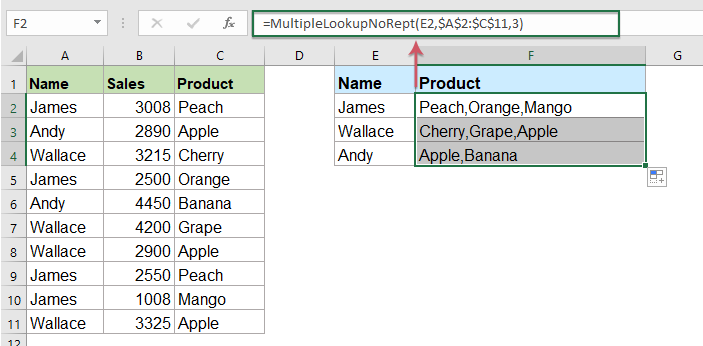Note: In the above formula, A2:C11 is the data range you want to use, E2 is the lookup value, the number 3 is the column number which contains the returned values.

#### Vlookup to return multiple values into one cell with a useful feature

If you have our Kutools for Excel, with its Advanced Combine Rows feature, you can quickly merge or combine the rows based on the same value and do some calculations as you need.

Note:To apply this Advanced Combine Rows, firstly, you should download the Kutools for Excel, and then apply the feature quickly and easily.

After installing Kutools for Excel, please do as follows:

1. Select the data range that you want to combine one column data based on another column.

2. Click Kutools > Merge & Split > Advanced Combine Rows, see screenshot: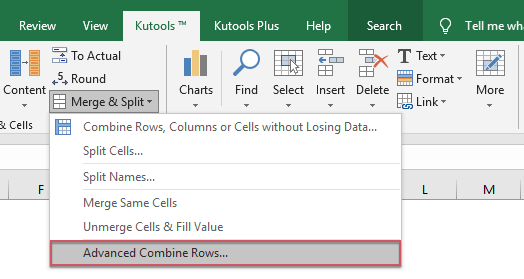3. In the popped out Advanced Combine Rows dialog box:

• Click the key column name to be combined based on, and then click Primary Key
• Then click another column that you want to combine its data based on the key column, and click Combine to choose one separator for separating the combined data.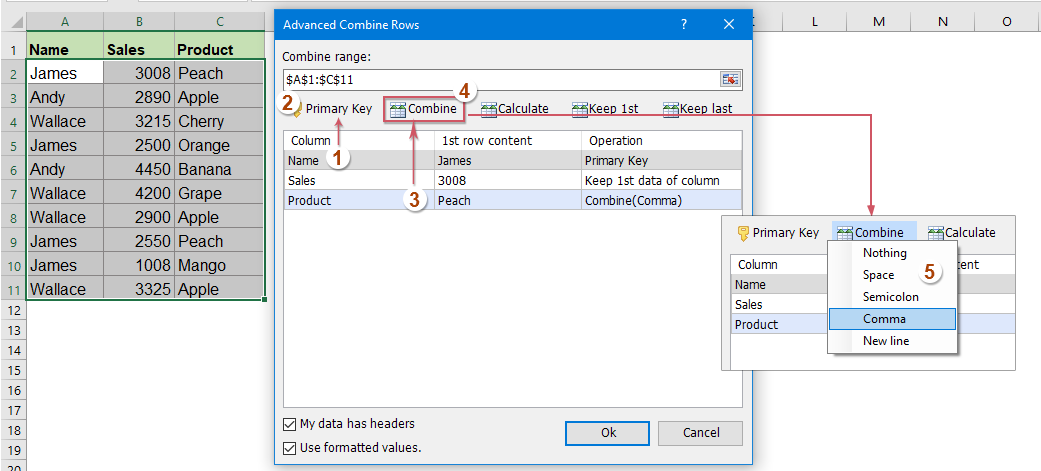4. Then click OK button, and you will get the following results: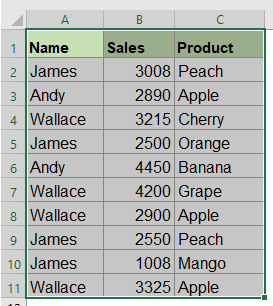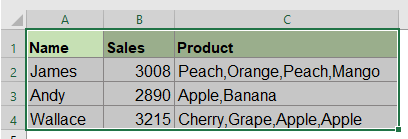#### More relative articles:

• VLOOKUP Function With Some Basic And Advanced Examples
• In Excel, the VLOOKUP function is a powerful function for most of Excel users, which is used to look for a value in the leftmost of the data range, and return a matching value in the same row from a column you specified. This tutorial is talking about how to use the VLOOKUP function with some basic and advanced examples in Excel.
• Return multiple matching values based on one or multiple criteria
• Normally, lookup a specific value and return the matching item is easy for most of us by using the VLOOKUP function. But, have you ever tried to return multiple matching values based on one or more criteria? In this article, I will introduce some formulas for solving this complex task in Excel.
• Vlookup And Return Multiple Values Vertically
• Normally, you can use the Vlookup function to get the first corresponding value, but, sometimes, you want to return all matching records based on a specific criterion. This article, I will talk about how to vlookup and return all matching values vertically, horizontally or into one single cell.
• Vlookup And Return Multiple Values From Drop Down List
• In Excel, how could you vlookup and return multiple corresponding values from a drop down list, which means when you choose one item from the drop down list, all of its relative values are displayed at once. This article, I will introduce the solution step by step.

### Best Office Productivity Tools

 Popular Features: Find, Highlight or Identify Duplicates   |  Delete Blank Rows   |  Combine Columns or Cells without Losing Data   |   Round without Formula ... Super Lookup: Multiple Criteria VLookup  |   Multiple Value VLookup  |   VLookup Across Multiple Sheets   |   Fuzzy Lookup .... Advanced Drop-down List: Quickly Create Drop Down List   |  Dependent Drop Down List   |  Multi-select Drop Down List .... Column Manager: Add a Specific Number of Columns  |  Move Columns  |  Toggle Visibility Status of Hidden Columns  |  Compare Ranges & Columns ... Featured Features: Grid Focus   |  Design View   |   Big Formula Bar   |  Workbook & Sheet Manager   |  Resource Library (Auto Text)   |  Date Picker   |  Combine Worksheets   |  Encrypt/Decrypt Cells   |  Send Emails by List   |  Super Filter   |   Special Filter (filter bold/italic/strikethrough...) ... Top 15 Toolsets:  12 Text Tools (Add Text, Remove Characters, ...)   |   50+ Chart Types (Gantt Chart, ...)   |   40+ Practical Formulas (Calculate age based on birthday, ...)   |   19 Insertion Tools (Insert QR Code, Insert Picture from Path, ...)   |   12 Conversion Tools (Numbers to Words, Currency Conversion, ...)   |   7 Merge & Split Tools (Advanced Combine Rows, Split Cells, ...)   |   ... and more

Supercharge Your Excel Skills with Kutools for Excel, and Experience Efficiency Like Never Before. Kutools for Excel Offers Over 300 Advanced Features to Boost Productivity and Save Time.  Click Here to Get The Feature You Need The Most...#### Office Tab Brings Tabbed interface to Office, and Make Your Work Much Easier

• Enable tabbed editing and reading in Word, Excel, PowerPoint, Publisher, Access, Visio and Project.
• Open and create multiple documents in new tabs of the same window, rather than in new windows.
• Increases your productivity by 50%, and reduces hundreds of mouse clicks for you every day!No ratings yet. Be the first to rate!
This comment was minimized by the moderator on the site
I have created a problem.
"I" have combined a "Textjoin" end "Vlookup" to return multiple values in to one single cell.
My problem is that the formula have to have an exact value to look for and I want it to lookup an "almost" match or Partial match.

Example: I have made a schedule how we ate going to work and a D1 is working from 07:30-16:00. And to lookup D1 is not the problem, the problem is that my boss sometimes puts other stuff togeather with the D1... Like "D1 +" or "D1 meeting".
Since my formula only lookup "D1" it misses for example the "D1 +".

My formula (that I have gotten from the web) =TEXTJOIN(" och ";SANT;OM(\$B\$3:\$B\$15=\$C\$22:\$F\$22;\$A\$3:\$A\$15;""))It´s in swedish so "SANT" is "TRUE" and "OM" is "IF".

How can I make the formula lookup all the cells that have some form of "D1" in it and return all those to the same cell?
No matter if it says "D1 +" or "D1 meeting" or whatever.
The reson I want this, is because the boss always leave "D1" but can add other text behind the "D1"... and just because of that, my boss messes up my formula.
This comment was minimized by the moderator on the site
Hi!
This is a great VBA-Code which could help me a lot.But when I start the Function MultipleLookupNoRept Excel crashs...I´ve got a Dataset with about 6.000 Rows (Excel 2013).... is this too much for the VBA Function?

Thanks!
This comment was minimized by the moderator on the site
Hello Mr.XXL,Sorry to hear that. The row limit for Excel 2013 is 1048576. Therefore, maybe the VBA code is the reason for the crash.
Anyway, I would love to offer you another VBA code for Vlookup To Return All Matching Values Without Duplicates Into One Cell. Please use the VBA code below:
Option Explicit

Function Lookup_concat(Search_string As String, _
Search_in_col As Range, Return_val_col As Range)

Dim i As Long
Dim temp() As Variant
Dim result As String
ReDim temp(0)

For i = 1 To Search_in_col.Count
If Search_in_col.Cells(i, 1) = Search_string Then
temp(UBound(temp)) = Return_val_col.Cells(i, 1).Value
ReDim Preserve temp(UBound(temp) + 1)
End If
Next

If temp(0) <> "" Then
ReDim Preserve temp(UBound(temp) - 1)
Unique temp
For i = LBound(temp) To UBound(temp)
result = result & " " & temp(i)
Next i
Lookup_concat = Trim(result)
Else
Lookup_concat = ""
End If

End Function

Function Unique(tempArray As Variant)

Dim coll As New Collection
Dim Value As Variant

On Error Resume Next
For Each Value In tempArray
If Len(Value) > 0 Then coll.Add Value, CStr(Value)
Next Value
On Error GoTo 0

ReDim tempArray(0)

For Each Value In coll
tempArray(UBound(tempArray)) = Value
ReDim Preserve tempArray(UBound(tempArray) + 1)
Next Value

End Function

After you insert this VBA code in the Module, please type the formula =Lookup_concat(E2,\$A\$2:\$A\$14,\$C\$2:\$C\$14) into a blank cell where you want to output the result, and then drag the fill hanlde down to get all matching values. Please see the file I uploaded in this comment. Hope it solves your problem.
Sincerely,Mandy

This comment was minimized by the moderator on the site
Hi, Thanks so much this worked!I used it to pull dates, that populated in the serial number format (<span style="letter-spacing: 0.2px; color: inherit; font-family: inherit; font-style: inherit; font-variant-ligatures: inherit; font-variant-caps: inherit; font-weight: inherit;">Changing the format to short date format using =TEXT(A2,”mm/dd/yy”) OR =DATEVALUE(A2) are not working. Do you have any solutions?</span>
This comment was minimized by the moderator on the site
Thank you for the explanations, however the function 'MultipleLookupNoRept' does not work on my file, could you tell me if an error exists.
This comment was minimized by the moderator on the site
Hi, Hasnae,Please check if you miss the third step -  check Microsoft Scripting Runtime option in the Available References list box.

This comment was minimized by the moderator on the site
Thank you so much for the code. Is there a way I can use the code to look up multiple values from multiple sheets? I tried to combine your function with IFERROR function but it doesn't seem to work.
This comment was minimized by the moderator on the site
Can this be modified to place the sum of those values? Instead of (400 400 400 400 400 400), can it sum them to show (2400)?
This comment was minimized by the moderator on the site
How with HLookUp function?
This comment was minimized by the moderator on the site
thanks for the code. I have modified it to allow you to optionally specify your own separator, Default is " ", if you specify the separator as"#cr" it will insert a CR/LF so the values will be on a separate line in the cell. It only applies the separator if there are multiple values

Function MYVLOOKUP(pValue As String, pWorkRng As Range, pIndex As Long, Optional ByVal pSep As Variant)

' ### Returns multiple values from a table into 1 cell ###

' pValue is the key value to lookup

' WorkRng is the Table you want to look up

' pIndex is the column # for the values to be returned from the pWorkRng

' pSep (optional) is the separator to be used. if omitted then default is a space (it doesn't apply the separator for the 1st entry)

' if the separtor = "#cr" it will separate the values on different line in the cell

Dim rng As Range

Dim sSep As String

Dim xResult As String

Dim Item1 As Boolean

Item1 = True

If IsMissing(pSep) = True Then

sSep = vbCr

Else

If pSep = "#cr" Then

sSep = vbCrLf

Else

sSep = pSep

End If

End If

xResult = ""

For Each rng In pWorkRng

If rng = pValue Then

If Item1 Then

xResult = xResult & rng.Offset(0, pIndex - 1)

Item1 = False

Else

xResult = xResult & sSep & rng.Offset(0, pIndex - 1)

End If

End If

Next

MYVLOOKUP = xResult

End Function
This comment was minimized by the moderator on the site
Thank you for this, the line breaks are what i needed to top this formula off! Question, is there a way to modify the code so that two values are compared? For example, similar to what we see with index and match, can i look for Product and Quantity columns, and based on those parameters it outputs results from the Region Column?
This comment was minimized by the moderator on the site
Thanks a lot for this code, it is very helpful. Does anyone know away to sum the values in the cell to just have at total of them.
Cheers
This comment was minimized by the moderator on the site
Hello, James, to sum values based on the corresponding items, the following article may help you, please chek it:
https://www.extendoffice.com/documents/excel/1268-excel-combine-duplicate-rows-and-sum.html
This comment was minimized by the moderator on the site
I have a server, it has connected with multiple applications. I want to compare compare two column and get the related applications details for that server.

What is the command for that.
There are no comments posted here yet